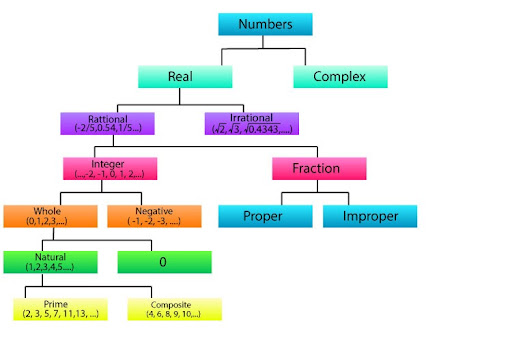# Write the smallest composite number and find the LCM of the smallest prime number and the smallest composite number.

In Mathematics , we have a variety of numbers varying from smallest numbers as 0 - 9 to largest numbers as 7896543.
These numbers are classified into different types, but first understand Why do we classify numbers? Why do we give them names, like integers, irrational numbers, or negative numbers? For the same reason we classify anything, we want to make sure that everyone has an understanding of what specific numbers are called and what they mean. After all, there’s a difference between 25, and -32, and 4⁶.
The different types of number are:
Whole numbers: Whole numbers are numbers we count with. 1, 2, 3, 4, and 5 are all whole numbers. So are -17 and 0. Whole numbers do not have fractions or decimals.
All whole numbers are called integers. Integers can be positive or negative whole numbers.
Natural numbers: Natural numbers are those numbers that are positive. So what about 0? 0 is considered as positive and negative both so it is not part of Natural numbers.
These Natural numbers are further classified into different types like EVEN numbers, ODD numbers, PRIME numbers and COMPOSITE numbers.
Here we need to discuss what are prime and composite numbers!
Prime numbers: The numbers that cannot be made by multiplying any two natural numbers. In simple words prime numbers are only divisible by themselves not any other number.
Let’s think of number 2! 2 is only divisible 1 and 2 itself. Same for 3 and 5. So the factors (the numbers by which it is divisible) are only two for Prime numbers: 1 and the number itself.
Composite numbers: Composite numbers are the numbers that can be divided exactly by a whole number other than itself.
Now, let's think 6 is a composite number or not?
6 can be exactly divided by 1 , 2 , 3 and 6 itself. So it has more factors which make it a composite number.
Solution:
Smallest Composite Number:

According to the classification of numbers, let’s check the numbers one by one.

1. 1 is considered neither Prime nor Composite number
2. 2 can be divided by 1 and 2 itself. Not a Composite number but the smallest Prime number.
3. 3 can be divided by 1 and 3 itself. Not a Composite number !
4. 4 can be divided by 1, 2 and 4 itself. So it’s a Composite number and the smallest Prime number.

So, 4 is the smallest Composite number.

LCM of the smallest Prime number and smallest Composite number:

LCM of two numbers is the least common multiple of the given two numbers.
We just found out that the smallest Prime number is 2 and the smallest Composite number is 4. So, the Least Common Multiple (LCM) for 2 and 4 can be found out by finding the factors of the numbers individually.

Factors of 2: 1, 2

Factors of 4: 1, 2 , 4

1 is not considered as a factor of any number so the least common multiple (LCM) is 2 for 2 and 4.